Maths-
General
Easy

Question

# The line among the following that touches the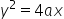is

##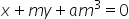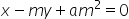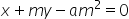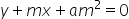Hint:

## The correct answer is:### Condition 1The line with slope 'm' is a tangent to parabolaThe equation of tangent to parabola is given as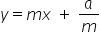(slope = m)Condition 2If slope =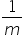, then equation of tangentThus, equation of lines touching parabola with slopeis.#### With Turito Foundation.#### Get an Expert Advice From Turito.